Worksheet: Can We Share

# Worksheet: Can We Share Notes | Study Mathematics for Class 3: NCERT - Class 3

## Document Description: Worksheet: Can We Share for Class 3 2022 is part of Mathematics for Class 3: NCERT preparation. The notes and questions for Worksheet: Can We Share have been prepared according to the Class 3 exam syllabus. Information about Worksheet: Can We Share covers topics like and Worksheet: Can We Share Example, for Class 3 2022 Exam. Find important definitions, questions, notes, meanings, examples, exercises and tests below for Worksheet: Can We Share.

Introduction of Worksheet: Can We Share in English is available as part of our Mathematics for Class 3: NCERT for Class 3 & Worksheet: Can We Share in Hindi for Mathematics for Class 3: NCERT course. Download more important topics related with notes, lectures and mock test series for Class 3 Exam by signing up for free. Class 3: Worksheet: Can We Share Notes | Study Mathematics for Class 3: NCERT - Class 3
 1 Crore+ students have signed up on EduRev. Have you?

Q.1. Fill in the blanks with the appropriate answer.
(i) 36 ÷ 6 = _____

(ii) 45 ÷ 9 = _____
(iii) 28 ÷ 4 = _____
(iv) 88 ÷ 2 = _____
(v) 63 ÷ _____ = 21
(vi) 48 ÷ 8 = ____
(vii) 56 ÷ 7 = _____
(viii) 244 ÷ _____ = 122
(ix) 99 ÷ 3 = _____
(x) 72 ÷  _____ = 9
(xi) 66 ÷ 2 = _____
(xii) 55 ÷ _____ = 5
(xiii) 81 ÷ _____ = 9
(xiv) 90 ÷ 9 = _____
(xv) 448 ÷ _____ = 112
(xvi) _____ ÷ 5 = 7
(xvii) 777 ÷ ____ = 7
(xviii) 120 ÷ 6 = _____
(xix) _____ ÷ 2 = 144
(xx) 49 ÷ _____ = 7

Q.2. I. Fill in the blanks:

 Multiplication fact Division fact 1 × 3 = ___ 3 ÷ 3 = ___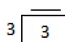2 × 3 = ___ 6 ÷ 3 = ___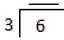3 × 3 = ___ 9 ÷ 3 = ___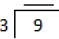4 × 3 = ___ 12 ÷ 3 = __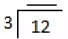5 × 3 = ___ 15 ÷ 3 = ___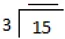6 × 3 = ___ 18 ÷ 3 = ___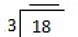7 × 3 = ___ 21 ÷ 3 = __ _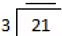8 × 3 = ___ 24 ÷ 3 = ___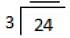9 × 3 = ___ 27 ÷ 3 = ___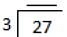10 × 3 = ___ 30 ÷ 3 = ___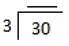II. Write two division facts for each multiplication fact:
(i) 6 × 3 = 18
(ii) 2 × 3 = 6
(iii) 9 × 3 = 27
(iv) 12 × 3 = 36
(v) 8 × 3 = 24

III. Write two multiplication facts for each division fact:
(i) 15 ÷ 3 = 5

(ii) 3 ÷ 3 = 1
(iii) 21 ÷ 3 = 7
(iv) 30 ÷ 3 = 10
(v) 33 ÷ 3 = 11

The document Worksheet: Can We Share Notes | Study Mathematics for Class 3: NCERT - Class 3 is a part of the Class 3 Course Mathematics for Class 3: NCERT.
All you need of Class 3 at this link: Class 3

## Mathematics for Class 3: NCERT

51 videos|84 docs|44 tests
 Use Code STAYHOME200 and get INR 200 additional OFF

## Mathematics for Class 3: NCERT

51 videos|84 docs|44 tests

Track your progress, build streaks, highlight & save important lessons and more!

,

,

,

,

,

,

,

,

,

,

,

,

,

,

,

,

,

,

,

,

,

;